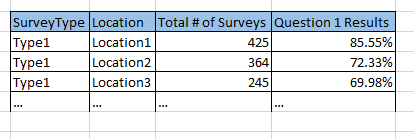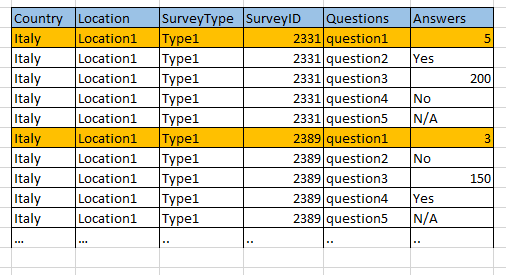# QlikView App Dev

Discussion Board for collaboration related to QlikView App Development.

Announcements
Now Live: Qlik Sense SaaS Simplified Authoring – Analytics Creation for Everyone: READ DETAILS
cancel
Showing results for
Did you mean:Contributor II

## Calculating the number of rows in a table without using Macro(from outside the table)

Note that this question is about getting the number of rows (distinct locations) of the report table using set analysis (displayed in a textbox)

My report looks like this – already working and validatedMy data consists of multiple line (5 for each survey)

Similar to this:The rules I need to apply are as following:

• Only question1 should be considered – for distinct surveys
• Question 1 has possible answers 1,2,3,4,5
• The above report is based on the results of Answers 5 for question1.
• The (number of 5 answered questions for a specific location) / (total number of surveys for that location) must be greater or equal to 0.63 (63%)
• And a location must have at least 8 surveys to be considered.

I got the Total # of surveys by using the following expression:

=If((Count({<Questions={'Question1'},Answers={'5'}>} distinct SurveyID) / Count({<Questions={'Question1'}>} distinct SurveyID))>=0.63 and Count(distinct SurveyID)>=8,Count(distinct SurveyID) )

And for  Question 1 Results I’m using:

=If(
(
Count({<Questions={'Question1'},Answers={'5'}>} distinct SurveyID)     / Count({<Questions={'Question1'}>} distinct SurveyID))>=0.63 and Count( distinct SurveyID)>=8
,
Count({<Questions={'Question1'},Answers={'5'}>} distinct SurveyID)     / Count({<Questions={'Question1'}>} distinct SurveyID))

The Above 2 expressions are giving the correct results for my data.

I’m having difficult in figuring out the number of rows (distinct locations) that are found in the report (basically number of rows) from outside the table displayed in a textbox.

1 Solution

Accepted SolutionsMVP

May be this

Sum(Aggr(

If((Count({<Questions={'Question1'},Answers={'5'}>} distinctSurveyID) / Count({<Questions={'Question1'}>} distinctSurveyID)) >= 0.63 and Count(DISTINCT SurveyID) >= 8, 1, 0)

, SurveyType, Location))

3 RepliesPartner - Champion III

Its not clear what you are asking for.

You said:

>>The Above 2 expressions are giving the correct results for my data.

and:

>>I’m having difficult in figuring out the number of rows...

Logic will get you from a to b. Imagination will take you everywhere. - A EinsteinContributor II
Author

Yes you are right

Let me put it in another way:

Assume that we have always SurveyType=Type1 and we are only interested in the results of question1.

Need to count the number of distinct locations where:

(Answered 5) / (total number of surveys for that location)  is >=0.63

and

the Location must have at least  8 surveys done there

does this help?

thank you againMVP

May be this

Sum(Aggr(

If((Count({<Questions={'Question1'},Answers={'5'}>} distinctSurveyID) / Count({<Questions={'Question1'}>} distinctSurveyID)) >= 0.63 and Count(DISTINCT SurveyID) >= 8, 1, 0)

, SurveyType, Location))